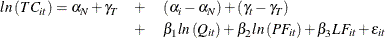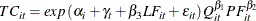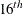The PANEL Procedure

Example 20.2 The Airline Cost Data: Fixtwo Model

The Christenson Associates airline data are a frequently cited data set (see Greene 2000). The data measure costs, prices of inputs, and utilization rates for six airlines over the time span 1970–1984. This example analyzes the log transformations of the cost, price and quantity, and the raw (not logged) capacity utilization measure. You speculate the following model:where theare the pure cross-sectional effects andare the time effects. The actual model speculated is highly nonlinear in the original variables. It would look like the following:The data and preliminary SAS statements are:

data airline;
input  Obs I T C Q PF LF;
label obs = "Observation number";
label I  = "Firm Number (CSID)";
label T  = "Time period (TSID)";
label Q  = "Output in revenue passenger miles (index)";
label C  = "Total cost, in thousands";
label PF = "Fuel price";
label LF = "Load Factor (utilization index)";
datalines;
1    1     1    1140640    0.95276     106650    0.53449

... more lines ...


data airline;
set airline;
lC = log(C);
lQ = log(Q);
lPF = log(PF);
label lC = "Log transformation of costs";
label lQ = "Log transformation of quantity";
label lPF= "Log transformation of price of fuel";
run;


The following statements fit the model.

proc panel data=airline printfixed;
id i t;
model lC = lQ lPF LF / fixtwo;
run;


First, you see the model’s description in Output 20.2.1. The model is a two-way fixed-effects model. There are six cross sections and fifteen time observations.

Output 20.2.1: The Airline Cost Data—Model Description

The PANEL Procedure
Fixed Two Way Estimates

Dependent Variable: lC Log transformation of costs

Model Description
Estimation Method FixTwo
Number of Cross Sections 6
Time Series Length 15

The R-square and degrees of freedom can be seen in Output 20.2.2. On the whole, you see a large R-square, so there is a reasonable fit. The degrees of freedom of the estimate are 90 minus 14 time dummy variables minus 5 cross section dummy variables and 4 regressors.

Output 20.2.2: The Airline Cost Data—Fit Statistics

Fit Statistics
SSE 0.1768 DFE 67
MSE 0.0026 Root MSE 0.0514
R-Square 0.9984

The F test for fixed effects is shown in Output 20.2.3. Testing the hypothesis that there are no fixed effects, you easily reject the null of poolability. There are group effects, or time effects, or both. The test is highly significant. OLS would not give reasonable results.

Output 20.2.3: The Airline Cost Data—Test for Fixed Effects

F Test for No Fixed Effects
Num DF Den DF F Value Pr > F
19 67 23.10 <.0001

Looking at the parameters, you see a more complicated pattern. Most of the cross-sectional effects are highly significant (with the exception of CS2). This means that the cross sections are significantly different from the sixth cross section. Many of the time effects show significance, but this is not uniform. It looks like the significance might be driven by a largeperiod effect, since the first six time effects are negative and of similar magnitude. The time dummy variables taper off in size and lose significance from time period 12 onward. There are many causes to which you could attribute this decay of time effects. The time period of the data spans the OPEC oil embargoes and the dissolution of the Civil Aeronautics Board (CAB). These two forces are two possible reasons to observe the decay and parameter instability. As for the regression parameters, you see that quantity affects cost positively, and the price of fuel has a positive effect, but load factors negatively affect the costs of the airlines in this sample. The somewhat disturbing result is that the fuel cost is not significant. If the time effects are proxies for the effect of the oil embargoes, then an insignificant fuel cost parameter would make some sense. If the dummy variables proxy for the dissolution of the CAB, then the effect of load factors is also not being precisely estimated.

Output 20.2.4: The Airline Cost Data—Parameter Estimates

Parameter Estimates
Variable DF Estimate Standard
Error
t Value Pr > |t| Label
CS1 1 0.174237 0.0861 2.02 0.0470 Cross Sectional Effect 1
CS2 1 0.111412 0.0780 1.43 0.1576 Cross Sectional Effect 2
CS3 1 -0.14354 0.0519 -2.77 0.0073 Cross Sectional Effect 3
CS4 1 0.18019 0.0321 5.61 <.0001 Cross Sectional Effect 4
CS5 1 -0.04671 0.0225 -2.08 0.0415 Cross Sectional Effect 5
TS1 1 -0.69286 0.3378 -2.05 0.0442 Time Series Effect 1
TS2 1 -0.63816 0.3321 -1.92 0.0589 Time Series Effect 2
TS3 1 -0.59554 0.3294 -1.81 0.0751 Time Series Effect 3
TS4 1 -0.54192 0.3189 -1.70 0.0939 Time Series Effect 4
TS5 1 -0.47288 0.2319 -2.04 0.0454 Time Series Effect 5
TS6 1 -0.42705 0.1884 -2.27 0.0267 Time Series Effect 6
TS7 1 -0.39586 0.1733 -2.28 0.0255 Time Series Effect 7
TS8 1 -0.33972 0.1501 -2.26 0.0269 Time Series Effect 8
TS9 1 -0.2718 0.1348 -2.02 0.0478 Time Series Effect 9
TS10 1 -0.22734 0.0763 -2.98 0.0040 Time Series Effect 10
TS11 1 -0.1118 0.0319 -3.50 0.0008 Time Series Effect 11
TS12 1 -0.03366 0.0429 -0.78 0.4354 Time Series Effect 12
TS13 1 -0.01775 0.0363 -0.49 0.6261 Time Series Effect 13
TS14 1 -0.01865 0.0305 -0.61 0.5430 Time Series Effect 14
Intercept 1 12.93834 2.2181 5.83 <.0001 Intercept
lQ 1 0.817264 0.0318 25.66 <.0001 Log transformation of quantity
lPF 1 0.168732 0.1635 1.03 0.3057 Log transformation of price of fuel
LF 1 -0.88267 0.2617 -3.37 0.0012 Load Factor (utilization index)